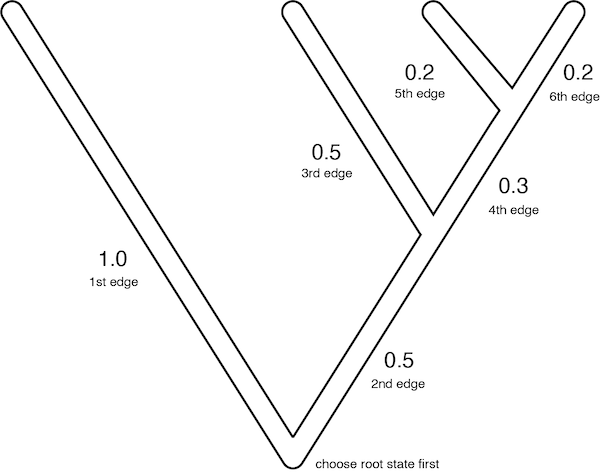Up to the Phylogenetics main page

## Simulation

Your challenge for this homework assignment is to simulate data for a single nucleotide site under an F81 model on the tree shown below.Assume that the nucleotide frequencies are:

$\pi_A=0.1, \pi_C=0.3, \pi_G=0.4, \pi_T=0.2$

The rate matrix for the F81 model is shown below (the ordering for both rows and columns is A, C, G, T):

$Q = \left( \begin{array}{cccc} -(1-\pi_A) \beta & \pi_C \beta & \pi_G \beta & \pi_T \beta \cr \pi_A \beta & -(1-\pi_C) \beta & \pi_G \beta & \pi_T \beta \cr \pi_A \beta & \pi_C \beta & -(1-\pi_G) \beta & \pi_T \beta \cr \pi_A \beta & \pi_C \beta & \pi_G \beta & -(1-\pi_T) \beta \end{array} \right)$

The edge lengths (in expected number of substitutions per site) are given on the tree.

You’ve been provided a tree topology with edge lengths, as well as values for the four base frequences, so all you need now to simulate a single DNA site on the tree provided is a few pseudorandom numbers.

import random
random.seed(12345)
for i in range(100):
u = random.random()
print('%0.3f' % u)


The little Python3 script above generates 100 pseudorandom numbers (you will not need to use all of these). Please use this program to draw your random numbers and use the random numbers in order (this will make it easy for me to check if you did things correctly because it should ensure that we all do the same thing). Note that the program spits out the numbers with 3 decimal places. Please round all numbers you use to 3 decimals places.

Your first random number (call it $u_1$) will be used to draw a starting state at the root of the tree. Use this table to decide which base should be at the root node:

state probability $u_1$ in range
A $\pi_A = 0.1$ 0.0 to 0.1
C $\pi_C = 0.3$ 0.1 to 0.4
G $\pi_G = 0.4$ 0.4 to 0.8
T $\pi_T = 0.2$ 0.8 to 1.0

Your second random number ($u_2$) will be used to draw a chunk of edge length using the formula below:

$-\log(1 - u_2)$

(Be sure to use natural logarithms; natural logarithm is often labeled ln on calculators). If you draw a chunk of edge length that is less than the length of an edge, then use the appropriate row of the rate matrix (and your next random number) to decide what state to substitute in at that point on the tree. For example, if the current state is A, the probability of changing to an G is

$\frac{\beta \pi_G}{\beta \pi_C + \beta \pi_G + \beta \pi_T} = \frac{\pi_G}{\pi_C + \pi_G + \pi_T}$

Noting that $\pi_C + \pi_G + \pi_T = 1 - \pi_A$ allows us to simplify this to

$\frac{\pi_G}{1 - \pi_A}$

To decide what state is left after the substitution event, create a table such as the following to make your decision:

state probability $u$ in range
C $\frac{\pi_C}{1-\pi_A} = 0.3/0.9$ 0.00000 to 0.33333
G $\frac{\pi_G}{1-\pi_A} = 0.4/0.9$ 0.33333 to 0.77778
T $\frac{\pi_T}{1-\pi_A} = 0.2/0.9$ 0.77778 to 1.00000

Note added in proof: The rows in tables like this should specify nucleotide states in alphabetical order: A, C, G, T. Using a different ordering will still yield a valid simulation, but homeworks will be much easier for me to check if we all do the same thing!

If the next random number $u$ is between 0.0 and 0.33333, then you would change the current state from A to C. If $u$ is between 0.33333 and 0.77778, you would change A to G. Finally, if $u$ is greater than 0.77778, you would change A to T. Simulate along the edges of the tree in the order indicated on the figure so that we will all get the same result. Remember that you don’t need to use excess chunks of edge length that extend beyond the node at the end of an edge. Once you determine the state at the far end of an edge, just start simulating from a clean slate using that state as your starting state for the next edge on the tree.

## What to turn in

Please color in the tree showing sojourns in different nucleotide states in different colors (or hatching patterns if you only have one color pen). (Here is a pdf version of the tree.) You can use colored pencils, different color pens, virtual ink on a tablet, or different kinds of stippling/striping/hatching to show sojourns, using a different color for A, C, G, and T. You need not be exact in the position of substitutions; just do your best to eyeball it (for example, if you decide a substitution should be at 0.32259 on a branch of length 1.0, placing the color change somewhere about a third of the way along the branch is fine).

Please also show how you determined your first sojourn time and how you determined the state that resulted from that substitution. Specify the random number drawn as well as the formulas used to determine the length of the sojourn and the state resulting from the substitution (a table like that shown above would be nice).